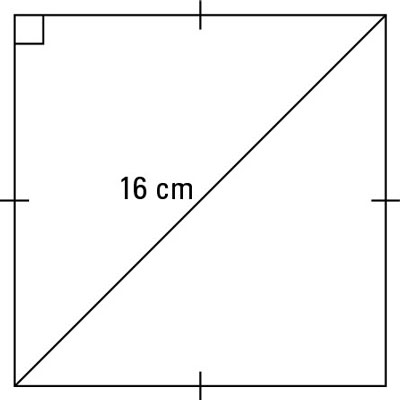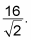Angles that have sides with a 45°/ 45°/90° usually have a unique ratio. The two legs are the exact same length, and the hypotenuse is that length times √2.

Whenever you are given a triangle as shown above – one where two of its angles are 45° and the third is 90°, you can easily figure out two of the sides. When you are asked to complete calculations with this type of triangle, it will fall into one of two categories:

• Type 1: You are given one leg.

Because you know both legs are equal, you know the length of both legs. You can find the hypotenuse by multiplying this length by the square root of 2.

• Type 2: You are given the hypotenuse.

Divide the hypotenuse by the square root of 2 to find the legs (which are equal).

Here’s an example calculation: The diagonal in a square is 16 centimeters long. How long is each side of the square? Draw it out first. The figure shows the square.The diagonal of a square divides the angles into 45° pieces, so you have the hypotenuse of a triangle as shown above. To find the legs, divide the hypotenuse by the square root of 2. When you do, you getYou must now rationalise the denominator to getwhich is the measure of each side of the square.

Source: dummies.com